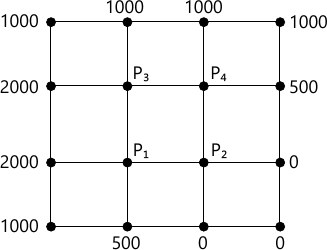MORE IN Engineering Mathematics 3
Total marks: --
Total time: --
INSTRUCTIONS
(1) Assume appropriate data and state your reasons
(2) Marks are given to the right of every question
(3) Draw neat diagrams wherever necessary

1 (a) Expand f(x)=x sin x as Fourier series in the interval (-π, π), hence deduce the following: $i) \ \dfrac {\pi}{2} = 1+ \dfrac {2}{1.3}- \dfrac {2}{3.5} + \dfrac {2}{5.7} \\ ii) \ \dfrac {\pi -2}{4} = \dfrac {1}{1.3} - \dfrac {1}{3.5}+ \dfrac {1}{5.7}+ \cdots \$
7 M
1 (b) Find the half-range Fourier cosine series for the function. $f(x)= \left\{\begin{matrix} kx &, \ 0\le x \le l/2 \\ k(l-x) &, \ l/2 6 M 1 (c) Find the constant term and the first two harmonics in the Fourier series for f(x) given by the following table:  x: 0 π/3 2π/3 π 4π/3 5π/3 2π F(x): 1 1.4 1.9 1.7 1.5 1.2 1.0 7 M 2 (a) Find the Fourier transform of the function f(x)=xe-h|x|. 7 M 2 (b) Find the Fourier sine transform of the \[ Function \ f(x)= \left\{\begin{matrix} \sin x &, \ 0 6 M 2 (c) Find the inverse Fourier sine Transform of \[ F_x (\alpha) = \dfrac {1} {\alpha} e^{-a\alpha} \ a>0.$
7 M

3 (a) Find various possible solution of one dimensional wave equation $\dfrac {\partial ^2 u}{\partial t^2} = C^2 \ \dfrac {\partial ^2 u}{\partial x^2}$ separable variable method.
7 M
3 (b) Obtain solution of heat equation $\dfrac {\partial u}{\partial t}= C^2 \dfrac {\partial ^2 u}{\partial t^2}$ subject to condition u(0, t)=0, u(l, t)=0, u(x,0)=f(x).
6 M
3 (c) Solve Laplace equation $\dfrac {\partial^2 u}{\partial x^2} + \dfrac {\partial^2 u}{\partial y^2}= 0$ subject to condition u(0,y)=u, u(x,0)=0. $u(x,a)= \sin \left ( \dfrac {\pi x}{l} \right )$
7 M

4 (a) The pressure P and volume V of a gas are related by the equation PVr=K, where r and K are constant. Find this equation to the following set of observations (in appropriate units)
 P: 0.5 1 1.5 2 2.5 3 V: 1.62 1 0.75 0.62 0.52 0.46
7 M
4 (b) Solve the following LPP using the Graphical method:
Maximize: Z=3x1+4x2
Under the constraints
4x1+2x2≤80
2x1+5x2≤180

x1, x2 ≥ 0
6 M
4 (c) Solve the following using simplex method,
Maximize: Z=2x+4y, subjected t the
Constraint: 3x+y ≤2z, 2x+3y≤24, x≥0, y≥0.
7 M

5 (a) Using the Regular-Falsi method, find a real root (correct to three decimal places) of the equation cos x=3x-1 that lies between 0.5 and 1 (Here, x is in radians).
7 M
5 (b) By relaxation method
Solve: x+6y+27z=85, 54x+y+z=110, 2x+15y+6z=72
6 M
5 (c) Using the power method, find the largest Eigen value and corresponding Eigen vectors of the matrix $A= \begin{bmatrix} 6 &-2 &2 \\-2 &3 &-1 \\2 &-1 &3 \end{bmatrix}$ taking [1, 1, 1]T as the initial Eigen vectors. Perform 5 iterations.
7 M

6 (a) From the data given in the following Table: find the number of students who obtained
(i) Less than 45 marks
(ii) between 40 and 45 marks
 Marks 30-40 40-50 50-60 60-70 70-80 No. of Students 31 42 51 35 31
7 M
6 (b) Using the Lagrange's formula, find the interpolating polynomial that approximetes to the function described by the following table:
 X 0 1 2 3 4 f(x) 3 6 11 18 27

Hence find f(0.5) and f(0.31)
6 M
6 (c) Evaluate $\int^1_0 \dfrac {x} {1+x^2} dx$ by using Simpson's (3/8)th Rule, dividing the interval into 3 equal parts. Hence find an approximate value of log √2.
7 M

7 (a) Solve the one-dimensional wave equation $\dfrac {\partial^2 u}{\partial x^2} = \dfrac {\partial^2 u}{\partial t^2}$ Subject to the boundary conditions u(0,t)=0, u(1, t)=0, t≤0 and the initial conditions $u(x,0)=\sin \pi x , \dfrac {\partial u}{\partial t} (x, 0) = 0, \ 0 < x < 1$
7 M
7 (b) Consider the heat equation $2\dfrac {\partial ^2 u}{\partial x^2} = \dfrac {\partial u}{\partial t}$ under the following condition.
i) u(0,t)=u (4,t)=0, t≥0.
ii) u(x,0)=x (4-x), 0 Employ the Bendre-Schmidt method with h=1 to find the solution of the equation for 0
6 M
7 (c) Solve the two-dimensional Laplace equation $\dfrac {\partial ^2 u}{\partial x^2}= \dfrac {\partial ^2 u}{\partial y^2}=0$ at the interior pivotal points of the square region shown in the following figure. The value of u at the pivotal points on the boundary are also shown in the figure.7 M

8 (a) State and prove the recurrence relation of Z-Transformation hence find ZT(np) and $Z_T \left [ \cos h \left (\dfrac {n \pi}{2}+0 \right ) \right ]$
7 M
8 (b) Find $Z_{T}^{-1} \left [ \dfrac {z^3 - 20 z}{(z-2)^3 (z-4)} \right ]$
6 M
8 (c) Solve the difference equation.
yn+2-2yn+1-3yn=3n+2n
Given y0=y1=0.
7 M

More question papers from Engineering Mathematics 3